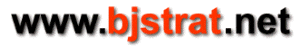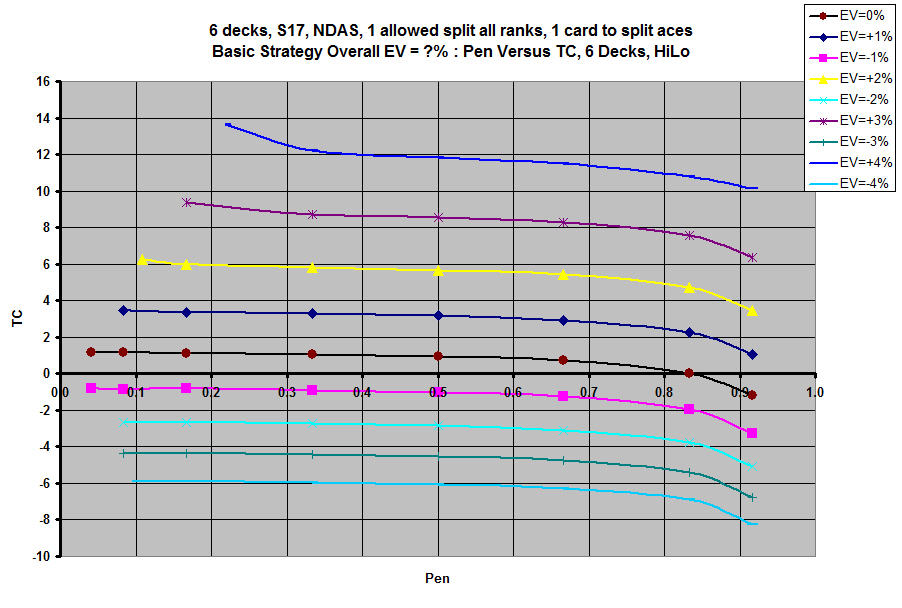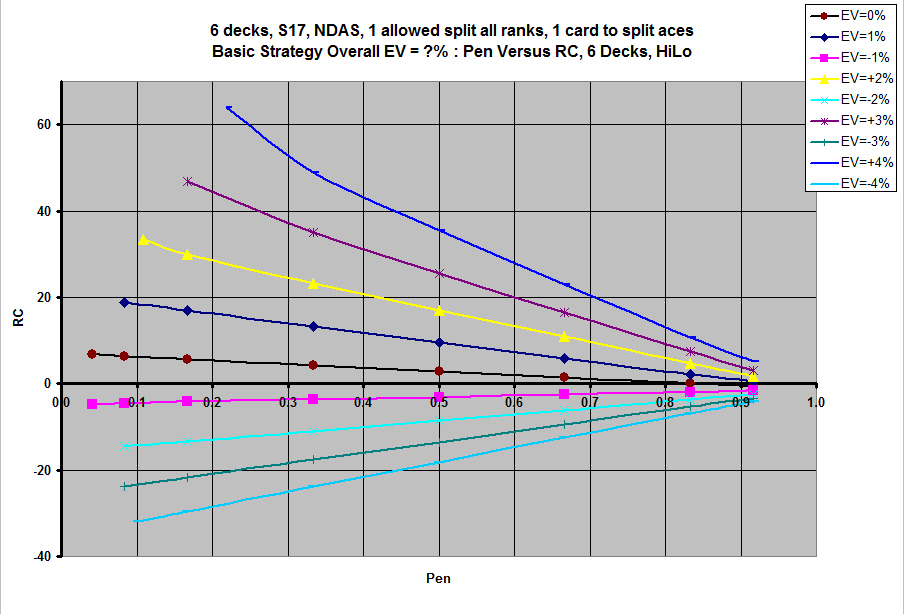An accepted method of referencing blackjack counting system variation is through a parameter known as true count. True count is dependent upon number of cards remaining to be dealt and the system's running count. Running count is simply the counting system's measure of imbalance between two or more groups of cards.

let CR = cards remaining
let RC = running count
let TC = true count

In general: TC = 52 * RC / CR

This method is usually used if a count is balanced.

Another method is to just simply use running count. This method is usually used if a count is unbalanced.

Either method could be used with exactly the same degree of precision when both options exist. However, the accepted use of true count is to assume a constant value. A plot of a constant true count on the vertical axis versus pen on the horizontal axis results in a horizontal line parallel to the horizontal axis. This simplification results in diminished precision to some extent. Check the true count versus pen plot for an estimate of variation from a constant horizontal value.

The point of this example is not to advocate one method or the other but just to show a measure of overall expected value using basic strategy for HiLo count using each. The exact same data is used to generate each plot.

### True Count Versus Pen### Running Count Versus Pen